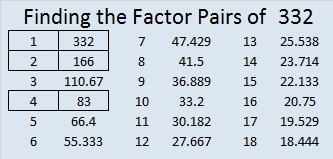# 332 and a Christmas Star

• 332 is a composite number.
• Prime factorization: 332 = 2 x 2 x 83 which can be written 2² x 83
• The exponents in the prime factorization are 1 and 1. Adding one to each and multiplying we get (2 + 1)(1 + 1) = 3 x 2 = 6. Therefore 332 has exactly 6 factors.
• Factors of 332: 1, 2, 4, 83, 166, 332
• Factor pairs: 332 = 1 x 332, 2 x 166, or 4 x 83
• Taking the factor pair with the largest square number factor, we get √332 = (√4)(√83) = 2√83 ≈ 18.221Merry Christmas! This is a rather easy level 5 puzzle so I’m sharing it instead of a level 4 puzzle today.Print the puzzles or type the factors on this excel file:  10 Factors 2014-12-22

The Night Wind excitedly asked a little lamb and the whole world, “Do you see what I see?” when it saw “a star, a star, dancing in the night” after the Christ child was born. Click here to read the complete lyrics of “Do You Hear What I Hear.”Advertisements